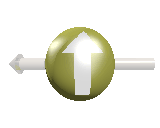So after a year and a half of work, I'm ready to release my paper, which might just revolutionise particle physics. To get it published is going to a fair effort with, no doubt, a lot of corrections and edits. Mean time i want to get a preprint out on ArXiv. So I'm asking for a physicist already preprinted there to give me an endorsement. And any feedback on my paper is welcome.

To read the paper, click herePDF FormatMy paper is reasonably technical, and aimed at publication in one of the physics journals, and thus is naturally full of mathematics. For less technically readers, and for physicists just scrim reading, I'll try and describe my idea as simply as possible here. So what i my idea? Briefly it is that: Just like electrons feel the electromagnetic force,  neutrinos have there own force that they feel.

Why should this be so? Well for several decades now, we'll had a good mathematical reason for the electromagnetic force to exist. Its due to an idea called local gauge invariance. Local gauge invariance is a symmetry, where by you change the quantum mechanical phase of a field of particles, by a different amount at every point in space-time. If you do this to an spin 1/2 particle, like a electron, then you automatically have to add to your theory a spin-1 force carrying particle, which lead to the electromagnetic force.

So what about the neutrino, that's another spin-1/2 particle. If you vary it's phase at each place in space time does that lead to a force? Well the answer depends on the neutrino's mass, specifical what type of mass it has. For ordinary particle, a left handing spinning particle has the same mass as a right hand spinning particle, this is known as Dirac type mass, after the English physics P.M. Dirac. But it doesn't look like neutrino have Dirac type mass, because they would be all those right handed neutrino states will the same microscopically small mass, as the left handed neutrino that we know about. And that would double the number of neutrino states during the Big Bang, which would upset, cosmologists wonderfully precise prediction of the the primordial abundances of the elements.

So neutrino mass must be of the other type, known as Majorana masses after the Italian physics, Ettore Majorana. So what happens if you try local guage invariance on a Majorana massed particle?. Well if there are  only left handed particles, it doesn't work at all, but if there are both left and right handed particle but with different masses, it looks like it does work. But it doesn't then produce a force like the electromagnetic force, which is forbidden, that Majorana mass type. Instead it a produces a force where:

<B>Left handed and Right neutrino neutrino's have opposite charges, under the new force.</b>

This turns out to be great at explaining how a particle (a state which is specific mass), can always be left handed. Because for a massive state spinning anti-clockwise, you can always accelerate, over take the particle, and look at it again form behind. And you'd then see it as spinning clockwise. Well that a state, that is not supposed it exist, and the mass of a particle of the force it feels shouldn't change depending on how fast you're moving. In fact that is a statement of Einsteins principle of relavity, that, the law of physics are the same to all observers no matter what velocity there travelling at. So what happens to our left handed neutrino when you over take it. In my model, it emits a spin-1, i call an axi-photon, which then lets it be viewed a left handed particle in the frame of  reference of the new observer. See the pictures.Imagine a neutrino, travelling to the right, and  spinning anticlockwise.

Now over take it, and look behind you. Its now travelling in the oppositedirection, but still spinning as before. But that is
a state that can't exist.In the Standard Model, instead a (Majorana Mass type) neutrino converts to an anti-neutrino, when you over take it. But this is odd, and violates Lepton Number Conversion. Often Physicists invoke a Spinless particle called a Majoron which converts the neutrino to an anti-neutrino.In the axial-force theory, the neutrino can instead reverse its spin at the same time as it reverses direction by emitted a axi-photon, which has Spin-1. So we get a fundamental process in which a neutrino reverses its spin and direction by emitting an axiphoton, as shown in the Feynman diiagram below.Great so this our force solves the mystery of neutrino spin. Now read on to page 2, where i explain, how it shows that the weak force has to be handed, what it means for quarks, why the proton has to be stable, and how my Axial force, may explain dark energy.

#### Now read page 2 to see why the weak force must be handedBy, Dr B.D.O Adams

See, My Blog Axitronics

for more on the axial force.

Links

See My Page on the Standard Model

Make a Free Website with Yola.